Vibroengineering PROCEDIA

Published: 19 October 2020

# 5-year monitoring of the dynamic properties of a 32-story SRC structure

Hui Tian1
Yinfeng Dong2
Dong Li3
1, 2, 3Key Laboratory of New Technology for Construction of cities in Mountain Area (Chongqing University), Ministry of Education, Chongqing, 400045, China
1, 2, 3School of Civil Engineering, Chongqing University, Chongqing, 400045, China
Corresponding Author:
Hui Tian
Views 65

#### Abstract

The dynamic properties of the structure are related to many factors, such as mass distribution, component stiffness, material properties. In addition, environmental conditions may also affect the dynamic characteristics. In this paper, the modal identification of 133 strong earthquakes recorded in a 32-story SRC building structure in Tokyo from 2013 to 2018 is performed first, and then the dynamic characteristics (natural frequency, damping ratio) are obtained. The statistical regression analysis is used to analyze the influence of environmental conditions (temperature, humidity) on the dynamic characteristics of the high-rise SRC building structure, and the change trend of its dynamic characteristics. Finally, the results of the analysis are given to provide experience and basis for the design of similar building structures.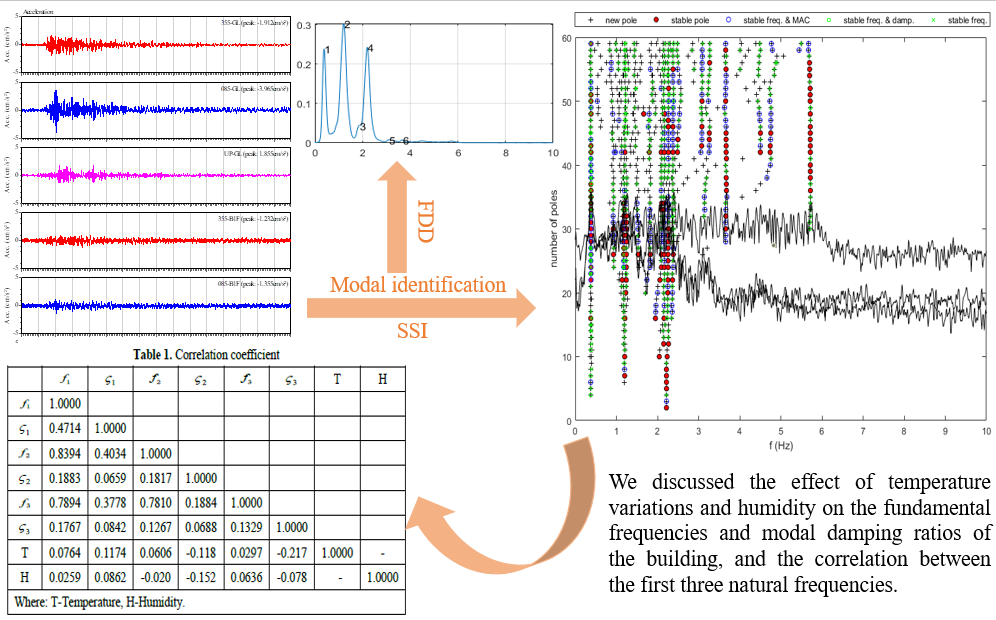#### Highlights

• This paper is mainly based on the FDD and SSI methods to obtain the dynamic characteristics of the structure, and uses relatively more stable results.
• The fluctuation range of the natural frequency and the damping ratio is obvious, the change range of the natural frequency reaches more than 30%, and the change range of the damping ratio is larger.
• The first three natural frequencies are more affected by temperature than relative humidity, while the corresponding first three damping ratios have the opposite result.
• The correlation between the first three fundamental frequencies is strong, indicating that the regular trends of fundamental frequencies are more consistent.

## 1. Introduction

With the rapid development of high-rise buildings, dynamic detection and structural health monitoring of high-rise building structures have become important issues. The response of a structure under earthquakes is closely related to the dynamic characteristics of the structure. The natural vibration period and damping ratio are the main dynamic characteristics of the building. The dynamic characteristics of the structure are related to many factors, such as mass distribution, component stiffness, material properties . In addition, environmental conditions  may also affect the dynamic characteristics. In this paper, firstly, the modal identification of the strong earthquake records of a 32-story SRC structure for 5 years is carried out, and the corresponding natural frequency and damping ratio are obtained. Then, through regression analysis, the impact of environmental factors (temperature, humidity) on its dynamic characteristics is revealed.

Fig. 1a) View of the building, b) aerial view from Maps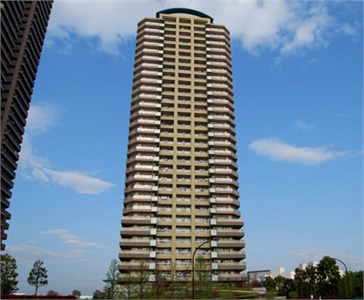a)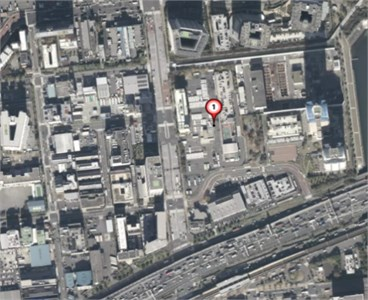b)

Fig. 2Strong motion records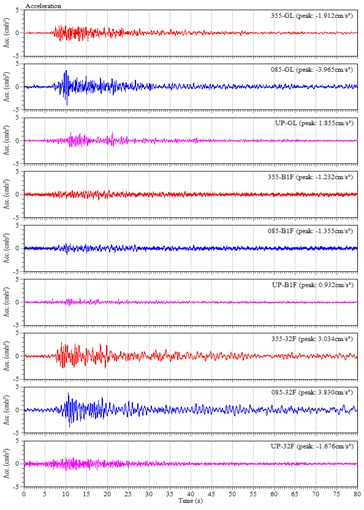## 2. Modal identification

Structural modal parameter identification is the process of obtaining the natural frequency, damping ratio and mode shape of the structure by using excitation, response or only output data. In structural health monitoring, this process is time varying. Structural modal parameter identification can be divided into experimental modal identification and operational modal identification; it can also be divided into frequency domain identification and time domain identification. This paper is mainly based on the frequency domain identification method of the Frequency Domain Decomposition (FDD) method and the time domain identification method of the Stochastic Subspace Identification (SSI) method to obtain the dynamic characteristics of the structure . Fig. 3 shows the typical identification results of the FDD method and SSI method.

Fig. 3a) Singular value decomposition plot, b) typical stabilization diagram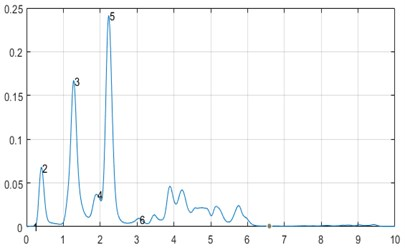a)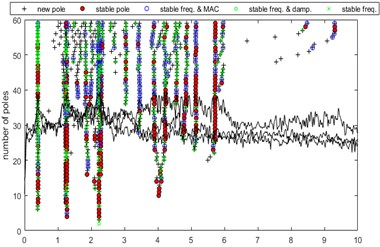b)

## 3. Results and discussions

### 3.1. 5-year change of dynamic characteristics

Fig. 4 shows the natural frequencies of the first three modes obtained from 133 seismic records from 2013 to 2018 based on the SSI method or the FDD method. The natural frequencies of the first three modes are approximately 0.39 Hz, 1.21 Hz, and 2.20 Hz, and the corresponding damping ratios are 3.30 %, 2.34 %, and 1.80 %, respectively. It is obvious that the fluctuation of damping ratios is larger than natural frequencies. Fig. 5 shows the power spectrum of natural frequencies and damping ratios Vs. time, the frequency contents of the change of first three natural frequencies and damping ratios with time are generally consistent. The trend of 2 year (0.5 cycle/year), 6 months (2 cycles/year) and 3 months (4~5 cycles/year) are clearly presented.

Fig. 4Modal parameter diagram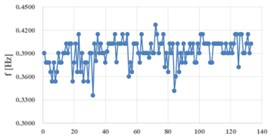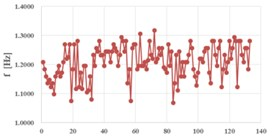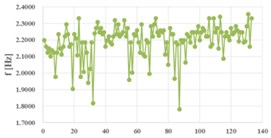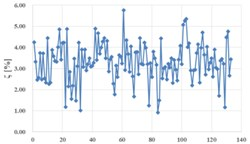a) 1st mode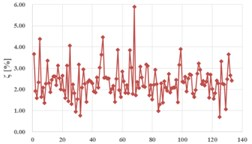b) 2nd mode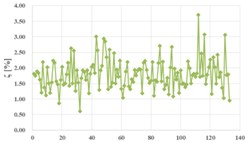c) 3rd mode

Fig. 5Power spectrum of natural frequencies and damping ratios Vs. time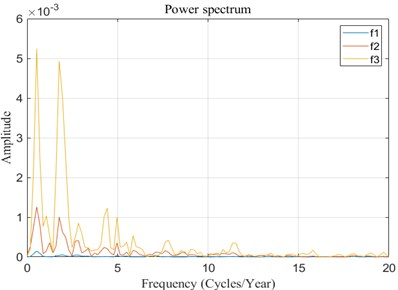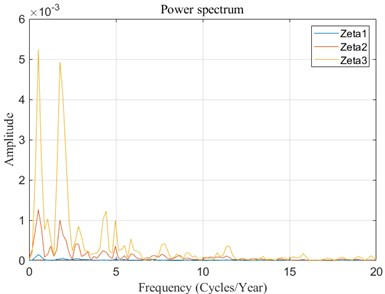### 3.2. The influence of temperature and humidity on dynamic characteristics

In general, according to Fig. 6 and 7, environmental conditions have a slight influence on the first three natural frequencies and damping ratios of the 32-story SRC building, especially the third natural frequency. The first three natural frequencies are more affected by temperature than relative humidity, while the corresponding first three damping ratios have the opposite result. The relevant data on the environmental conditions used here is obtained from website of Japan Meteorological Agency (http://www.jma.go.jp/jma/index.html).

### 3.3. Correlation analysis

The correlation coefficients between the natural frequencies ${f}_{1}$, ${f}_{2}$, and ${f}_{3}$ are 0.8394, 0.7894, 0.7810, respectively. Table 1 and Fig. 8 also show the strong correlation between the natural frequencies. The correlation between natural frequency and damping ratio and environmental factors is not obvious, which is consistent with the small slope in Figs. 6 and 7.

Fig. 6The influence of temperature on natural frequency and damping ratio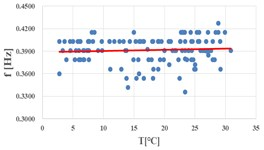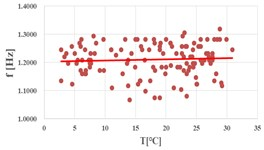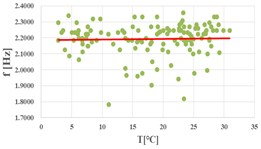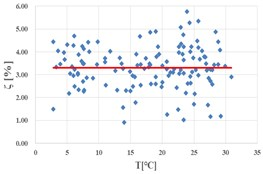a) 1st mode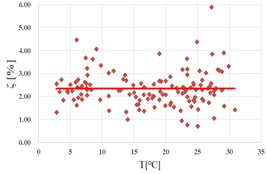b) 2nd mode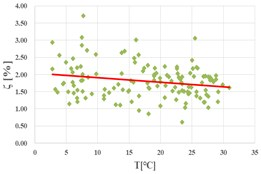c) 3rd mode

Fig. 7The influence of relative humidity on dynamic characteristics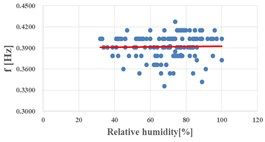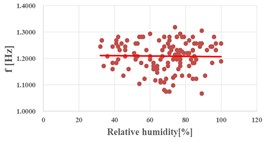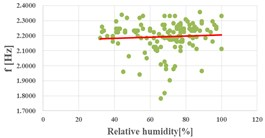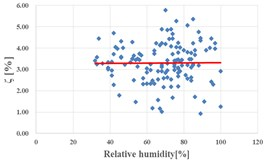a) 1st mode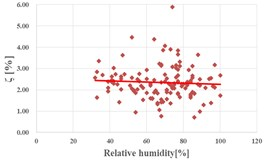b) 2nd mode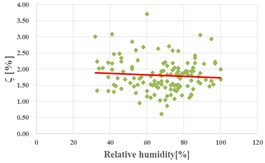c) 3rd mode

Fig. 8Correlation between natural frequencies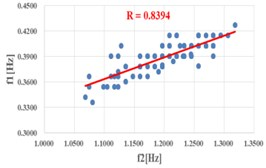a)${f}_{1}$${f}_{2}$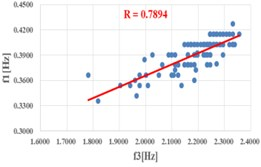b)${f}_{1}$${f}_{3}$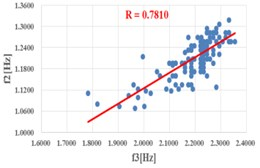c)${f}_{2}$${f}_{3}$

Table 1Correlation coefficient

 ${f}_{1}$ ${\varsigma }_{1}$ ${f}_{2}$ ${\varsigma }_{2}$ ${f}_{3}$ ${\varsigma }_{3}$ $T$ $H$ ${f}_{1}$ 1.0000 ${\varsigma }_{1}$ 0.4714 1.0000 ${f}_{2}$ 0.8394 0.4034 1.0000 ${\varsigma }_{2}$ 0.1883 0.0659 0.1817 1.0000 ${f}_{3}$ 0.7894 0.3778 0.7810 0.1884 1.0000 ${\varsigma }_{3}$ 0.1767 0.0842 0.1267 0.0688 0.1329 1.0000 $T$ 0.0764 0.1174 0.0606 –0.118 0.0297 –0.217 1.0000 – $H$ 0.0259 0.0862 –0.020 –0.152 0.0636 –0.078 – 1.0000 Where: $T$ – Temperature, $H$ – humidity

## 4. Conclusions

The dynamic characteristics of this 32-story SRC building were obtained through modal identification recorded by 133 strong motion records. We discussed the effect of temperature variations and humidity on the fundamental frequencies and modal damping ratios of the building, and the correlation between the first three natural frequencies.

1) The natural frequencies of the first three modes are approximately 0.39 Hz, 1.21 Hz, and 2.20 Hz, and the corresponding damping ratios are 3.30 %, 2.34 %, and 1.80 %, respectively. The damping ratio results of modal identification are all less than 5 %, and the higher the modal, the smaller the corresponding damping ratio. The fluctuation range of the natural frequency and the damping ratio is obvious, the change range of the natural frequency reaches more than 30 %, and the change range of the damping ratio is larger.

2) According to the results of regression analysis, environmental conditions have tiny obvious influence on the dynamic characteristics of the SRC building. The results also show that the fluctuations of the first three natural frequencies and damping ratios are caused by other factors, and temperature and humidity are not the main reasons.

3) The correlation between the first three fundamental frequencies is strong, indicating that the regular trends of fundamental frequencies are more consistent.

#### Acknowledgements

We thank the BRI Strong Motion Network of Japan (https://smo.kenken.go.jp/) for providing access to the data used in this study. The temperature and humidity data comes from the website of Japan Meteorological Agency (http://www.jma.go.jp/jma/index.html).

#### Cited by

27 September 2020
Accepted
13 October 2020
Published
19 October 2020
SUBJECTS
Seismic engineering and applications
Keywords
SRC
strong motion records
modal identification
environmental conditions
dynamic properties
influence
regression analysis